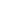Flat 50% off

Ends in

• JEE Main & Advanced
•• 11th
•• Physics
• Back to Doubt Clearing

## A small block of mass m is fixed at upper end of a massless vertical spring of spring constant K = 4mg/L and natural length '10L'. The lower end of spring is free and is at a height L from fixed horizontal floor. The spring is initially unstressed and the spring-block system is released from rest.When free end of spring just touches the ground, the velocity of the block at that instant is 'v'. Then which of the following is (are) true :(a) The magnitude of velocity 'v' is $\sqrt {2gL}$.(b) Block wil regain the velocity of magnitude 'v', when compression in spring is $\frac {L}{2}$.(c) Block will reach the velocity of magnitude 'v', twice in a cycle.(d) Block will reach the velocity of magnitude 'v', four times in a cycle.

### Asked By abc k

Updated Tue, 11 Dec 2018 03:39 pm